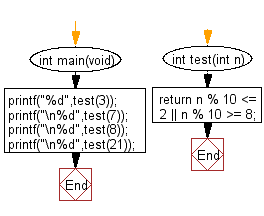﻿ C Program: If a number is within 2 of a multiple of 10# C Exercises: Check whether a given number is within 2 of a multiple of 10

## C-programming basic algorithm: Exercise-21 with Solution

Write a C program to check whether a given number is within 2 of a multiple of 10.

C Code:

``````#include <stdio.h>
#include <stdlib.h>

int main(void){
printf("%d",test(3));
printf("\n%d",test(7));
printf("\n%d",test(8));
printf("\n%d",test(21));
}

int test(int n)
{
return n % 10 <= 2 || n % 10 >= 8;
}
``````

Sample Output:

```0
0
1
1
```

Flowchart:C Programming Code Editor:

What is the difficulty level of this exercise?

Test your Programming skills with w3resource's quiz.

﻿

## C Programming: Tips of the Day

Why doesn't a+++++b work?

printf("%d",a+++++b); is interpreted as (a++)++ + b according to the Maximal Munch Rule!.

++ (postfix) doesn't evaluate to an lvalue but it requires its operand to be an lvalue.

! 6.4/4 says the next preprocessing token is the longest sequence of characters that could constitute a preprocessing token"

Ref : https://bit.ly/3fdldUT